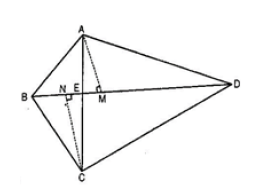# Diagonals AC and BD of a quadrilateral ABCD intersect each other at P. Show that ar (APB) ×ar (CPD) = ar (APD) ×ar (BPC) . [Hint : From A and C, draw perpendiculars to BD.]Solution:

Given:

The diagonal AC and BD of the quadrilateral ABCD, intersect each other at point E.

Construction:

From A, draw AM perpendicular to BD

From C, draw CN perpendicular to BD

To Prove

ar(ΔAED) ar(ΔBEC) = ar (ΔABE) ×ar (ΔCDE)

L.H.S. ar.(AEB)X(LED)

½ X EB X AM X 1/2 X DE X CN ————-(i)

¼ X EB X AM X DE X LN

R.H.S. ar.(AED)X ar.(BEC)

½ X DE X AM X 1/2 X BE X CN

¼ X DE X AM X BE X CN

¼ X EB X AM X DE X CN ————-(ii)

From 1 and 2

L.H.S=R.H.S

∴ar.(AEB)Xar.(CED)=ar.(AED)Xar.(BEC)

Hence Proved